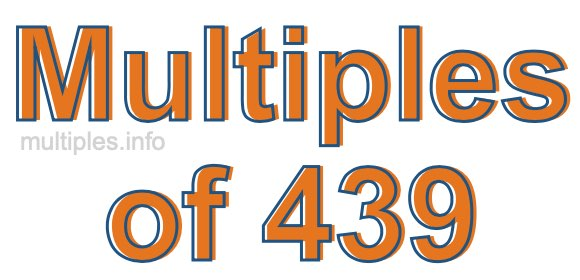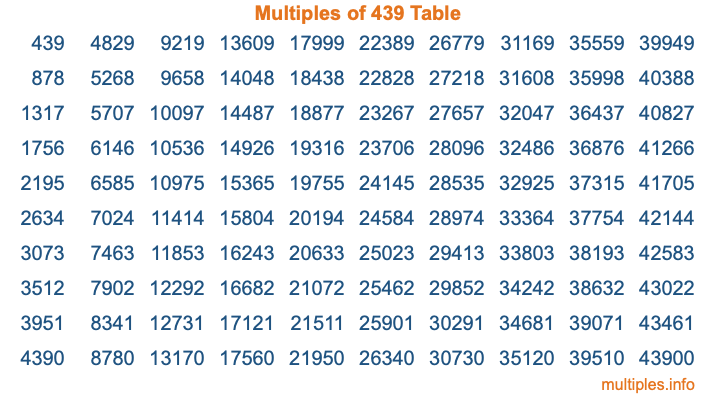Multiples of 439Welcome to the Multiples of 439 page. Here we will first teach you everything you will ever need to know about the multiples of 439, and then give you a study guide summary of everything we taught you to make sure you remember it all. Use this page to look up facts and learn information about the multiples of 439. This page will make you a multiples of four hundred thirty-nine expert!

Definition of Multiples of 439
Multiples of 439 are all the numbers that when divided by 439 equal an integer. Each of the multiples of 439 are called a multiple. A multiple of 439 is created by multiplying 439 by an integer.

Therefore, to create a list of multiples of 439, you start with 1 multiplied by 439, then 2 multiplied by 439, then 3 multiplied by 439, and so on for as long as you want. Thus, the list of the first five multiples of 439 is 439, 878, 1317, 1756, and 2195. To see a larger list of multiples of 439, see the printable image of Multiples of 439 further down on this page. We also have a category where you can choose any nth multiple of 439.

Multiples of 439 Checker
The Multiples of 439 Checker below checks to see if any number of your choice is a multiple of 439. In other words, it checks to see if there is any number (integer) that when multiplied by 439 will equal your number. To do that, we divide your number by 439. If the the quotient is an integer, then your number is a multiple of 439.

Is  a multiple of 439?

Least Common Multiple of 439 and ...
A Least Common Multiple (LCM) is the lowest multiple that two or more numbers have in common. This is also called the smallest common multiple or lowest common multiple and is useful to know when you are adding our subtracting fractions. Enter one or more numbers below (439 is already entered) to find the LCM.

Check out our LCM Calculator if you need more details about the Least Common Multiple or if you need the LCM for different numbers for adding and subtraction fractions.

nth Multiple of 439
As we stated above, 439 is the first multiple of 439, 878 is the second multiple of 439, 1317 is the third multiple of 439, and so on. Enter a number below to find the nth multiple of 439.

th multiple of 439

Multiples of 439 vs Factors of 439
439 is a multiple of 439 and a factor of 439, but that is where the similarities end. All postive multiples of 439 are 439 or greater than 439. All positive factors of 439 are 439 or less than 439.

Below is the beginning list of multiples of 439 and the factors of 439 so you can compare:

Multiples of 439: 439, 878, 1317, 1756, 2195, etc.

Factors of 439: 1, 439

As you can see, the multiples of 439 are all the numbers that you can divide by 439 to get a whole number. The factors of 439, on the other hand, are all the whole numbers that you can multiply by another whole number to get 439.

It's also interesting to note that if a number (x) is a factor of 439, then 439 will also be a multiple of that number (x).

Multiples of 439 vs Divisors of 439
The divisors of 439 are all the integers that 439 can be divided by evenly. Below is a list of the divisors of 439.

Divisors of 439: 1, 439

The interesting thing to note here is that if you take any multiple of 439 and divide it by a divisor of 439, you will see that the quotient is an integer.

Multiples of 439 Table
Below is an image of the first 100 multiples of 439 in a table. The table is in chronological order, column by column. The first column has the first ten multiples of 439, the second column has the next ten multiples of 439, and so on.The Multiples of 439 Table is also referred to as the 439 Times Table or Times Table of 439. You are welcome to print out our table for your studies.

Negative Multiples of 439
Although not often discussed or needed in math, it is worth mentioning that you can make a list of negative multiples of 439 by multiplying 439 by -1, then by -2, then by -3, and so on, to get the following list of negative multiples of 439:

-439, -878, -1317, -1756, -2195, etc.

Multiples of 439 Summary
Below is a summary of important Multiples of 439 facts that we have discussed on this page. To retain the knowledge on this page, we recommend that you read through the summary and explain to yourself or a study partner why they hold true.

There are an infinite number of multiples of 439.

A multiple of 439 divided by 439 will equal a whole number.

439 divided by a factor of 439 equals a divisor of 439.

The nth multiple of 439 is n times 439.

The largest factor of 439 is equal to the first positive multiple of 439.

439 is a multiple of every factor of 439.

439 is a multiple of 439.

A multiple of 439 divided by a divisor of 439 equals an integer.

439 divided by a divisor of 439 equals a factor of 439.

Any integer times 439 will equal a multiple of 439.

Multiples of a Number
Here you can get the multiples of another number, all with the same attention to detail as we did for multiples of 439 on this page.

Multiples of
Multiples of 440
Did you find our page about multiples of four hundred thirty-nine educational? Do you want more knowledge? Check out the multiples of the next number on our list!